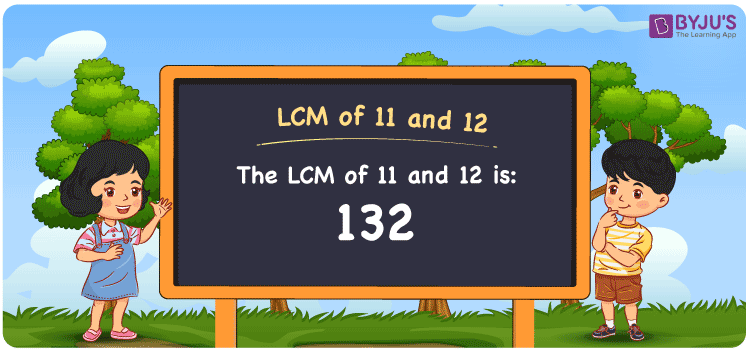Checkout JEE MAINS 2022 Question Paper Analysis : Checkout JEE MAINS 2022 Question Paper Analysis :

# LCM of 11 and 12

LCM of 11 and 12 is 132. From the multiples of 11 and 12, the number evenly divisible by the two numbers is the LCM value. Least common multiple of 11 and 12 is the common multiple we get using the multiplication operation. (11, 22, 33, 44, 55, ….) and (12, 24, 36, 48, 60, ….) are the multiples of 11 and 12. The LCM of two numbers is found by using methods such as listing multiples, prime factorization and division.

## What is LCM of 11 and 12?

The answer to this question is 132. The LCM of 11 and 12 using various methods is shown in this article for your reference. The LCM of two non-zero integers, 11 and 12, is the smallest positive integer 132 which is divisible by both 11 and 12 with no remainder.## How to Find LCM of 11 and 12?

LCM of 11 and 12 can be found using three methods:

• Prime Factorisation
• Division method
• Listing the multiples

### LCM of 11 and 12 Using Prime Factorisation Method

The prime factorisation of 11 and 12, respectively, is given by:

11 = 11¹

12 = 2 x 2 x 3 = 2² x 3¹

LCM (11, 12) = 132

### LCM of 11 and 12 Using Division Method

We’ll divide the numbers (11, 12) by their prime factors to get the LCM of 11 and 12 using the division method (preferably common). The LCM of 11 and 12 is calculated by multiplying these divisors.

 2 11 12 2 11 6 3 11 3 11 11 1 x 1 1

No further division can be done.

Hence, LCM (11, 12) = 132

### LCM of 11 and 12 Using Listing the Multiples

To calculate the LCM of 11 and 12 by listing out the common multiples, list the multiples as shown below

 Multiples of 11 Multiples of 12 11 12 22 24 33 36 44 48 55 60 66 72 77 84 88 96 99 108 110 120 121 132 132 –

LCM (11, 12) = 132

## Video Lesson on Applications of LCM## LCM of 11 and 12 Solved Examples

Find the smallest number which is exactly divisible by 11 and 12.

Solution:

We know that;

LCM is the smallest number exactly divisible by 11 and 12.

Multiples of 11 = 11, 22, 33, 44, 55, 66, ….

Multiples of 12 = 12, 24, 36, 48, 60, 72, …..

Hence, the LCM of 11 and 12 is 132.

## Frequently Asked Questions on LCM of 11 and 12

### List the methods used to determine the LCM of 11 and 12.

The methods used to determine the LCM of 11 and 12 are

Prime Factorisation

Division method

Listing the multiples

### Using the prime factorisation method, what is the LCM of 11 and 12?

We should analyze the factors first to determine the LCM

11 = 11¹

12 = 2 x 2 x 3 = 2² x 3¹

LCM is the product of prime factors raised to the highest exponent among 11 and 12.

LCM of 11 and 12 = 132

### Calculate the GCF if the LCM of 11 and 12 is 132.

LCM x GCF = 11 x 12

As the LCM = 132

132 x GCF = 132

GCF = 132/132 = 1